Author: Oscar Cronquist Article last updated on February 12, 2018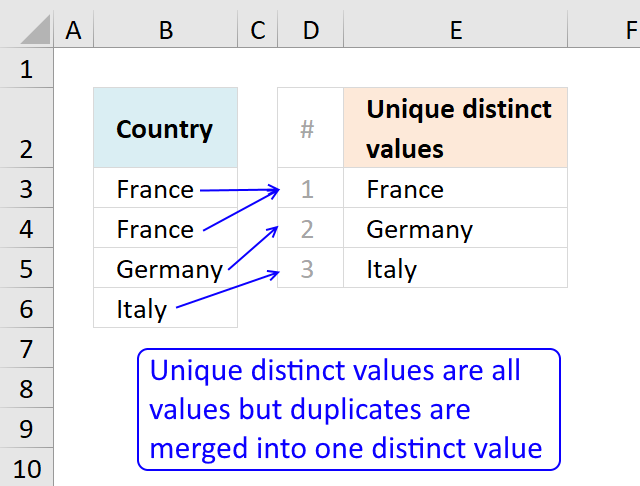This article describes how to count unique distinct values. What are unique distinct values? They are all values but duplicates are merged into one distinct value.

You will also find a formula to count unique values, see the table of Contents below. Unique values are values that exist only once in a list or cell range. If a value has a duplicate they are not unique and not counted.

If you are working with lots of data I highly recommend using a pivot table. Excel 2013 and later versions allow you to count unique distinct values.

### Count unique distinct values

The total number of unique distinct values are calculated in cell D3. The formula is not case sensitive, in other words, value FRANCE is the same as france.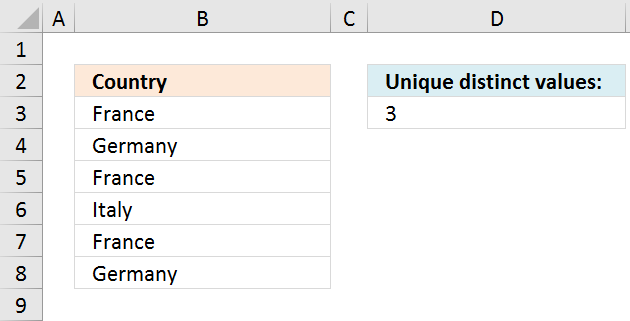Formula in cell E3:

=SUMPRODUCT(1/COUNTIF(\$B\$3:\$B\$8, \$B\$3:\$B\$8))

Recommended article:

Count unique distinct values based on a condition

The following article demonstrates how to construct a formula that counts unique distinct values based on a condition. The image […]

#### Watch a video where I explain the formula

The following formula is an array formula although slightly smaller than the regular formula above, however, you need to enter it as an array formula.

=SUM(1/COUNTIF(\$B\$3:\$B\$8, \$B\$3:\$B\$8))

How to enter an array formula

Keep in mind that if you have a blank in your cell range the formulas above won't work, read this: Count unique distinct values in a cell range with blanks

#### How the formula works

=SUMPRODUCT(1/COUNTIF(\$B\$3:\$B\$8, \$B\$3:\$B\$8))

Step 1 - Count each value

COUNTIF(\$B\$3:\$B\$8, \$B\$3:\$B\$8)

becomes

COUNTIF({"France"; "Germany"; "France"; "Italy"; "France"; "Germany"}, {"France"; "Germany"; "France"; "Italy"; "France"; "Germany"})

and returns {3, 2, 3, 1, 2, 3}. This array is shown in column C on the picture below.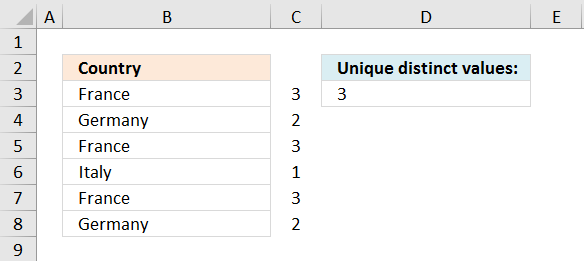How to use the COUNTIF function

Counts the number of cells that meet a specific condition.

Step 2 - Divide 1 with array

1/COUNTIF(\$B\$3:\$B\$8, \$B\$3:\$B\$8)

becomes

1/ {3, 2, 3, 1, 3, 2}

and returns {1/3, 1/2, 1/3, 1, 1/3, 1/2} This array is shown in column C on the picture below.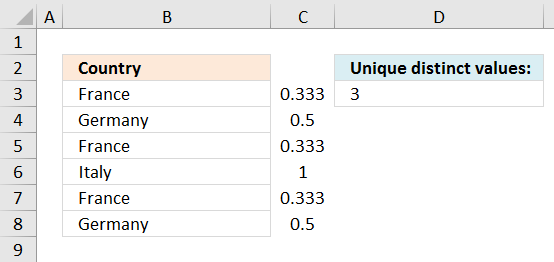Step 3 - Sum values

SUMPRODUCT(1/COUNTIF(\$B\$3:\$B\$8, \$B\$3:\$B\$8))

becomes

SUMPRODUCT({1/3, 1/2, 1/3, 1, 1/2, 1/3})

and returns 3.

1/3 + 1/2 + 1/3 + 1/1 + 1/2 + 1/3 = 3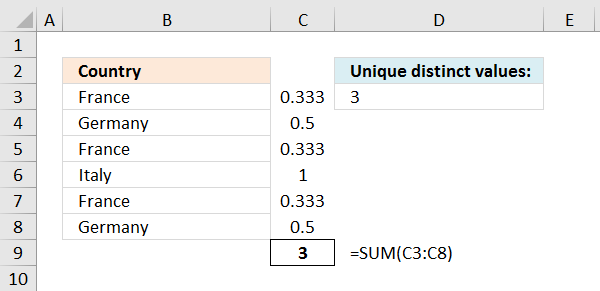How to use the SUM function

The SUM function in Excel allows you to add values, the function returns the sum in the cell it is […]

### Count unique distinct values in a cell range with blanks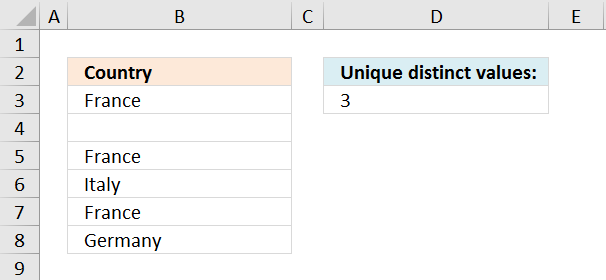Array formula in cell D3:

=SUM(IF(\$B\$3:\$B\$8<>"",1/COUNTIF(\$B\$3:\$B\$8, \$B\$3:\$B\$8), 0))

Watch a youtube video where I explain the formula

Alternative array formula

=COUNT(1/(--MATCH(\$B\$3:\$B\$8,\$B\$3:\$B\$8,0)=MATCH(ROW(\$B\$3:\$B\$8),ROW(\$B\$3:\$B\$8))))

Recommended article

Count unique distinct records

The image above shows a table with 3 columns containing random data. It is quite complicated trying to manually count […]

#### How to create an array formula

1. Copy (Ctrl + c) and paste (Ctrl + v) array formula into formula bar.
2. Press and hold Ctrl + Shift.
3. Press Enter once.
4. Release all keys.

How to enter an array formula

Array formulas allows you to do advanced calculations not possible with regular formulas.

count-unique-distinct-values-in-a-column.xls
(Excel 97-2003 Workbook *.xls)

### Count unique distinct values (case sensitive)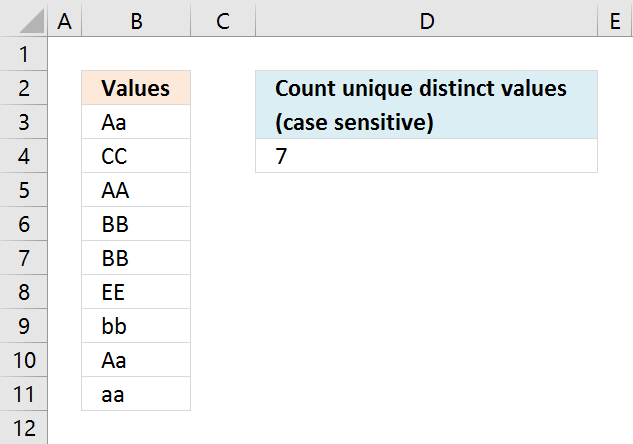Array formula in cell D4:

=SUM(1/MMULT(EXACT(\$B\$3:\$B\$11, TRANSPOSE(\$B\$3:\$B\$11))*1, ROW(\$B\$3:\$B\$11)^0))

#### Watch a video where I explain the formula

Recommended article

Extract unique distinct values (case sensitive) [Formula]

5 easy ways to extract Unique Distinct Values

First, let me explain the difference between unique values and unique distinct values, it is important you know the difference […]

#### How to enter an array formula

1. Select cell C2
2. Click in formula bar
3. Paste above array formula
4. Press and hold Ctrl + Shift
5. Press Enter

#### Explaining array formula in cell D4

Step 1 - Compare values against each other using the EXACT function

EXACT(\$B\$3:\$B\$11, TRANSPOSE(\$B\$3:\$B\$11))

becomes

=EXACT({"Aa"; "CC"; "AA"; "BB"; "BB"; "EE"; "bb"; "Aa"; "aa"}, {"Aa", "CC", "AA", "BB", "BB", "EE", "bb", "Aa", "aa"})

and returns the following boolean array, shown in picture below. Boolean values are TRUE or FALSE.

I have added the original values horizontally and vertically, they are also bolded.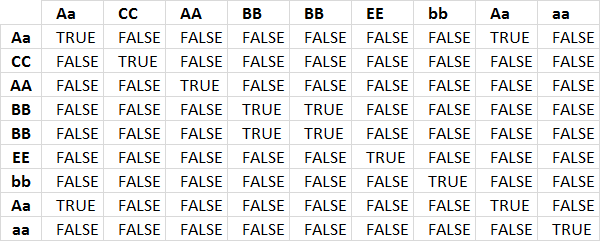The first column shows that value Aa exists twice because there are two TRUE in the first row.

Step 2 - Add values row-wise

The MMULT function allows you to add numbers for each row. To be able to do that I need to convert the boolean values to integers, in this case 0 or 1.

The MMULT function needs two arguments, the second argument must be an array of 1's with the same number of rows as the array in the first argument.

MMULT(EXACT(\$B\$3:\$B\$11, TRANSPOSE(\$B\$3:\$B\$11))*1, ROW(\$B\$3:\$B\$11)^0)

becomes

=MMULT({1, 0, 0, 0, 0, 0, 0, 1, 0; 0, 1, 0, 0, 0, 0, 0, 0, 0; 0, 0, 1, 0, 0, 0, 0, 0, 0; 0, 0, 0, 1, 1, 0, 0, 0, 0; 0, 0, 0, 1, 1, 0, 0, 0, 0; 0, 0, 0, 0, 0, 1, 0, 0, 0; 0, 0, 0, 0, 0, 0, 1, 0, 0; 1, 0, 0, 0, 0, 0, 0, 1, 0; 0, 0, 0, 0, 0, 0, 0, 0, 1}, {1; 1; 1; 1; 1; 1; 1; 1; 1})

and returns {2; 1; 1; 2; 2; 1; 1; 2; 1}. This array is shown in the column to the right.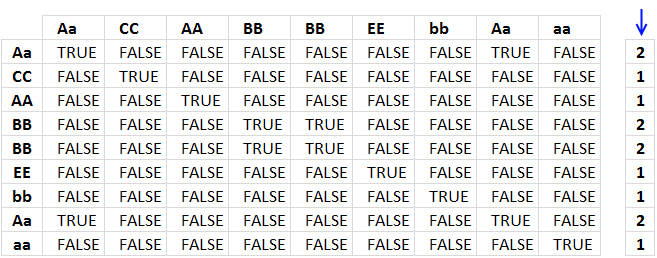Step 3 - Divide 1 with array

1/MMULT(EXACT(\$B\$3:\$B\$11, TRANSPOSE(\$B\$3:\$B\$11))*1, ROW(\$B\$3:\$B\$11)^0)

returns {0.5; 1; 1; 0.5; 0.5; 1; 1; 0.5; 1}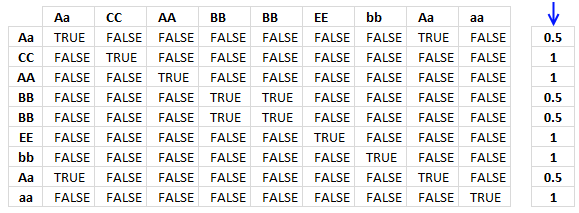Step 4 - Sum values

=SUM(--(MMULT(EXACT(\$B\$3:\$B\$11, TRANSPOSE(\$B\$3:\$B\$11))*1, ROW(\$B\$3:\$B\$11)^0)=1))

becomes

=SUM({0.5; 1; 1; 0.5; 0.5; 1; 1; 0.5; 1})

and returns 7 in cell D4.

Count unique distinct values case sensitive.xlsx

### Count unique values in a column

Unique values are values that exist only once, see picture below.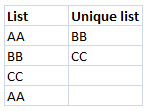AA has a duplicate and is not unique. BB and CC have no duplicates and are unique.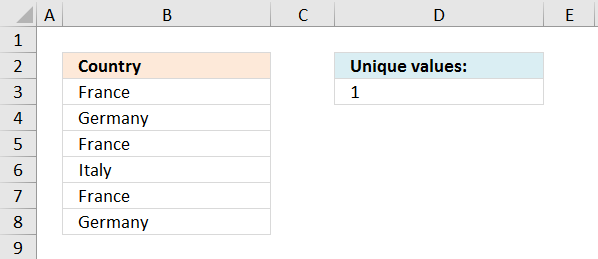Formula in D3:

=SUMPRODUCT(--(COUNTIF(\$B\$3:\$B\$8,\$B\$3:\$B\$8)=1))

There is only one unique value in the list (Italy), all other values have duplicates.

#### Explaining formula in cell C3

Step 1 - Count all values

The COUNTIF function counts how many values in a cell range that match a condition. In this case, the second argument has multiple values and this makes the COUNTIF function return an array of values.

COUNTIF(\$B\$3:\$B\$8,\$B\$3:\$B\$8)

becomes

COUNTIF({"France"; "Germany"; "France"; "Italy"; "France"; "Germany"},{"France"; "Germany"; "France"; "Italy"; "France"; "Germany"})

and returns the array: {3; 2; 3; 1; 3; 2}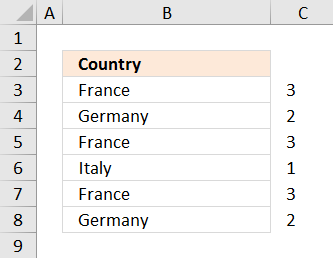This array tells us that the value France exists three times in cell range B3:B8. Germany exists twice and Italy is a unique value meaning there is only one instance of Italy in cell range B3:B8.

How to use the COUNTIF function

Counts the number of cells that meet a specific condition.

Step 2 - Check each value is equal to 1

COUNTIF(\$B\$3:\$B\$8,\$B\$3:\$B\$8)=1

becomes

{3; 2; 3; 1; 3; 2}=1

and returns {FALSE; FALSE; FALSE; TRUE; FALSE; FALSE}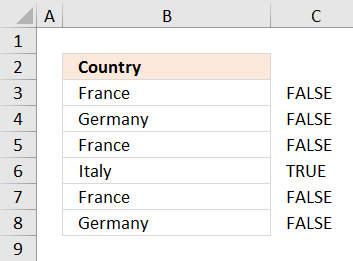Step 3 - Convert boolean values to integers

To be able to sum the boolean values I need to convert them to their equivalent 0 and 1. TRUE is 1 and FALSE is 0.

--(COUNTIF(\$B\$3:\$B\$8,\$B\$3:\$B\$8)=1)

becomes

--({FALSE; FALSE; FALSE; TRUE; FALSE; FALSE})

and returns {0; 0; 0; 0; 0; 0; 1; 0; 0}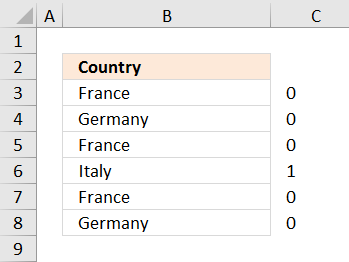Step 4 - Sum values

=SUMPRODUCT(--(COUNTIF(\$B\$3:\$B\$8,\$B\$3:\$B\$8)=1))

becomes

=SUMPRODUCT({0; 0; 0; 0; 0; 0; 1; 0; 0})

and returns 1 in cell D3.count-unique-distinct-values-in-a-column.xls
(Excel 97-2003 Workbook *.xls)

#### Recommended blog posts:

Count unique distinct records in Excel

### Count unique values (case sensitive)

The picture below shows values in column B, the formula in cell D3 counts 5 unique values in column B.

They are CC, AA, EE, bb and aa. They only exist once in column B.

Aa and BB have duplicates and are not unique.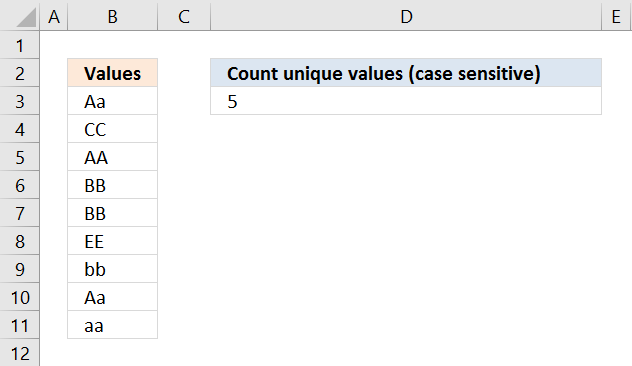Array formula in cell D3:

=SUM(--(MMULT(EXACT(\$B\$3:\$B\$11, TRANSPOSE(\$B\$3:\$B\$11))*1, ROW(\$B\$3:\$B\$11)^0)=1))

How to enter an array formula

#### Explaining array formula in cell D3

Step 1 - Compare values against each other using the EXACT function

EXACT(\$B\$3:\$B\$11, TRANSPOSE(\$B\$3:\$B\$11))

becomes

=EXACT({"Aa"; "CC"; "AA"; "BB"; "BB"; "EE"; "bb"; "Aa"; "aa"}, {"Aa", "CC", "AA", "BB", "BB", "EE", "bb", "Aa", "aa"})

and returns the following boolean array, shown in picture below. Boolean values are TRUE or FALSE.

I have added the original values horizontally and vertically, they are also bolded.The first column shows that value Aa exists twice because there are two TRUE in the first row.

Step 2 - Add values row-wise

The MMULT function allows you to add numbers for each row. To be able to do that I need to convert the boolean values to integers, in this case 0 or 1.

The MMULT function needs two arguments, the second argument must be an array of 1's with the same number of rows as the array in the first argument.

MMULT(EXACT(\$B\$3:\$B\$11, TRANSPOSE(\$B\$3:\$B\$11))*1, ROW(\$B\$3:\$B\$11)^0)

becomes

=MMULT({1, 0, 0, 0, 0, 0, 0, 1, 0; 0, 1, 0, 0, 0, 0, 0, 0, 0; 0, 0, 1, 0, 0, 0, 0, 0, 0; 0, 0, 0, 1, 1, 0, 0, 0, 0; 0, 0, 0, 1, 1, 0, 0, 0, 0; 0, 0, 0, 0, 0, 1, 0, 0, 0; 0, 0, 0, 0, 0, 0, 1, 0, 0; 1, 0, 0, 0, 0, 0, 0, 1, 0; 0, 0, 0, 0, 0, 0, 0, 0, 1}, {1; 1; 1; 1; 1; 1; 1; 1; 1})

and returns {2; 1; 1; 2; 2; 1; 1; 2; 1}. This array is shown in the column to the right.Step 3 - Check if values in array are equal to 1

MMULT(EXACT(\$B\$3:\$B\$11, TRANSPOSE(\$B\$3:\$B\$11))*1, ROW(\$B\$3:\$B\$11)^0)=1

returns {FALSE; TRUE; TRUE; FALSE; FALSE; TRUE; TRUE; FALSE; TRUE}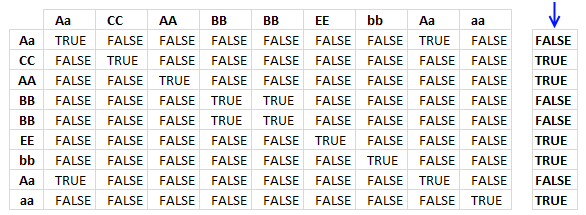Step 4 - Convert boolean values to integers

The SUM function can't add boolean values, to be able to do that I need to convert boolean values to integers.

--(MMULT(EXACT(\$B\$3:\$B\$11, TRANSPOSE(\$B\$3:\$B\$11))*1, ROW(\$B\$3:\$B\$11)^0)=1)

returns {0;1;1;0;0;1;1;0;1}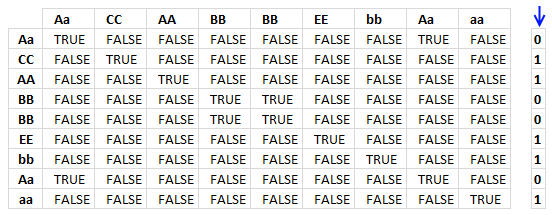Step 5 - Sum values

=SUM(--(MMULT(EXACT(\$B\$3:\$B\$11, TRANSPOSE(\$B\$3:\$B\$11))*1, ROW(\$B\$3:\$B\$11)^0)=1))

becomes

=SUM({0;1;1;0;0;1;1;0;1})

and returns 5 in cell D3.